# RD Sharma Solutions for Class 8 Math Chapter 3 - Squares and Square Roots (Part-7) Notes | Study RD Sharma Solutions for Class 8 Mathematics - Class 8

## Class 8: RD Sharma Solutions for Class 8 Math Chapter 3 - Squares and Square Roots (Part-7) Notes | Study RD Sharma Solutions for Class 8 Mathematics - Class 8

The document RD Sharma Solutions for Class 8 Math Chapter 3 - Squares and Square Roots (Part-7) Notes | Study RD Sharma Solutions for Class 8 Mathematics - Class 8 is a part of the Class 8 Course RD Sharma Solutions for Class 8 Mathematics.
All you need of Class 8 at this link: Class 8

####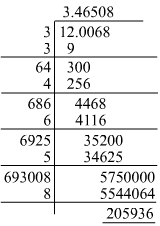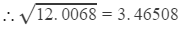We can round it off to four decimal places, i.e. 3.4651.

#### Answer 3: Using the long division method: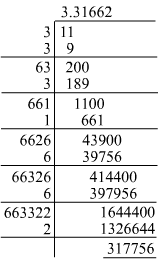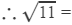3.31662

#### Question 4: Given that: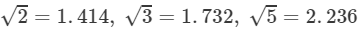and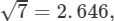evaluate each of the following: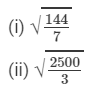#### Answer 4: Given: √7 = 2.646

(i)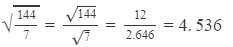Given: √3 = 1.732

(ii)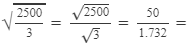28.867(ii) 25003=25003=501.732=28.867

#### Question 5: Given that: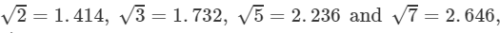find the square roots of the following: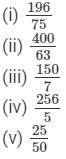#### Answer 5: From the given values, we can simplify the expressions in the following manner:

(i)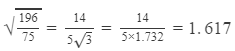(ii)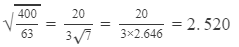(iii)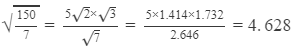(iv)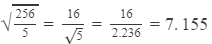(v)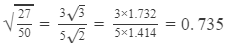#### Answer 7: Using the table to find √3 and √5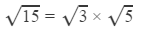=1.732×2.236=3.873

#### Answer 8: Using the table to find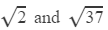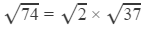=1.414×6.083

=8.602

#### Answer 9: Using the table to find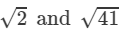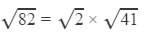=1.414×6.403

=9.055

#### Answer 10: Using the table to find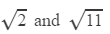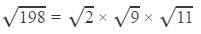=1.414×3×3.317

= 14.070

#### Answer 11: Using the table to find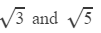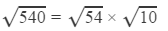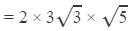=2×3×1.732×2.2361

=23.24

#### Answer 12: Using the table to find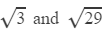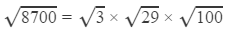=1.7321×5.385×10

=93.27

#### Answer 13: Using the table to find √29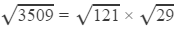=11×5.3851

=59.235

#### Answer 14: Using the table to find √14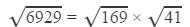=13 ×6.4031

=83.239

#### Answer 15: Using the table to find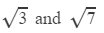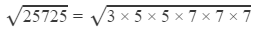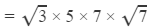=1.732 ×5×7×2.646

=160.41

#### Answer 16: Using the table to find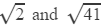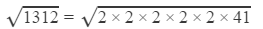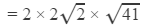=2×2×1.414×6.4031

=36.222

#### Answer 17: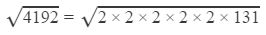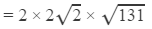The square root of 131 is not listed in the table. Hence, we have to apply long division to find it.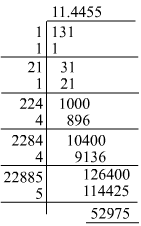Substituting the values:

=   2×2×11.44552×2×11.4455     (using the table to find2)

= 64.75

#### Answer 18: On prime factorisation:4955 is equal to 5 ×× 991, which means that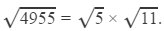The square root of 991 is not listed in the table; it lists the square roots of all the numbers below 100.
Hence, we have to manipulate the number such that we get the square root of a number less than 100. This can be done in the following manner: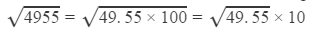Now, we have to find the square root of 49.55.

We have: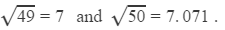Their difference is 0.071.
Thus, for the difference of 1 (50 - 49), the difference in the values of the square roots is 0.071.
For the difference of 0.55, the difference in the values of the square roots is:
0.55 ×× 0.0701 = 0.03905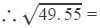7+0.03905=7.03905

Finally, we have: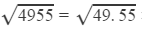×10=7.03905×10=70.3905

####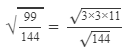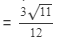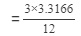(using the square root table to find √11)

=0.829

####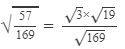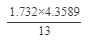(using the square root table to find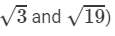0.581

#### Question 21: Using square root table, find the square root 101/169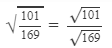The square root of 101 is not listed in the table. This is because the table lists the square roots of all the numbers below 100.
Hence, we have to manipulate the number such that we get the square root of a number less than 100. This can be done in the following manner: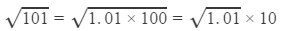Now, we have to find the square root of 1.01.

We have: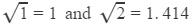Their difference is 0.414.
Thus, for the difference of 1 (2 - 1), the difference in the values of the square roots is 0.414.
For the difference of 0.01, the difference in the values of the square roots is:
0.01 ×× 0.414 = 0.00414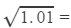1+0.00414=1.00414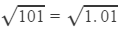×10=1.00414×10=10.0414
Finally,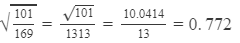This value is really close to the one from the key answer.

#### Answer 22: From the square root table, we have: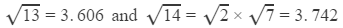Their difference is 0.136.
Thus, for the difference of 1 (14 - 13), the difference in the values of the square roots is 0.136.
For the difference of 0.21, the difference in the values of their square roots is:
0.136×0.21=0.028560.136×0.21=0.02856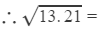3.606+0.02856≈3.635

#### Answer 23:We have to find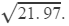From the square root table, we have: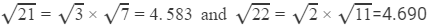Their difference is 0.107.
Thus, for the difference of 1 (22 - 21), the difference in the values of the square roots is 0.107.
For the difference of 0.97, the difference in the values of their square roots is:
0.107×0.97=0.1040.107×0.97=0.104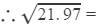4.583+0.104≈4.687

####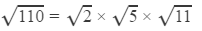=1.414×2.236×3.317        (Using the square root table to find all the square roots)

=10.488

####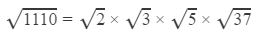=1.414×1.732×2.236×6.083       (Using the table to find all the square roots )=33.312

#### 11.11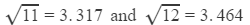Their difference is 0.1474.
Thus, for the difference of 1 (12 - 11), the difference in the values of the square roots is 0.1474.
For the difference of 0.11, the difference in the values of the square roots is:
0.11 ×× 0.1474 = 0.0162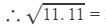3.3166+0.0162=3.328≈3.333

#### Answer 27:The length of one side of the square field will be the square root of 325.∴∴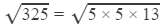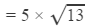=5×3.605

=18.030

Hence, the length of one side of the field is 18.030 m.

#### Answer 28: The area of the rectangle = 240 m ×× 70 m = 16800 m2Given that the area of the square is equal to the area of the rectangle.Hence, the area of the square will also be 16800 m2.The length of one side of a square is the square root of its area.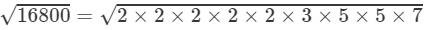=2×2×5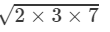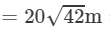=129.60 m

Hence, the length of one side of the square is 129.60 m

The document RD Sharma Solutions for Class 8 Math Chapter 3 - Squares and Square Roots (Part-7) Notes | Study RD Sharma Solutions for Class 8 Mathematics - Class 8 is a part of the Class 8 Course RD Sharma Solutions for Class 8 Mathematics.
All you need of Class 8 at this link: Class 8Use Code STAYHOME200 and get INR 200 additional OFF

## RD Sharma Solutions for Class 8 Mathematics

88 docs

Track your progress, build streaks, highlight & save important lessons and more!

,

,

,

,

,

,

,

,

,

,

,

,

,

,

,

,

,

,

,

,

,

;